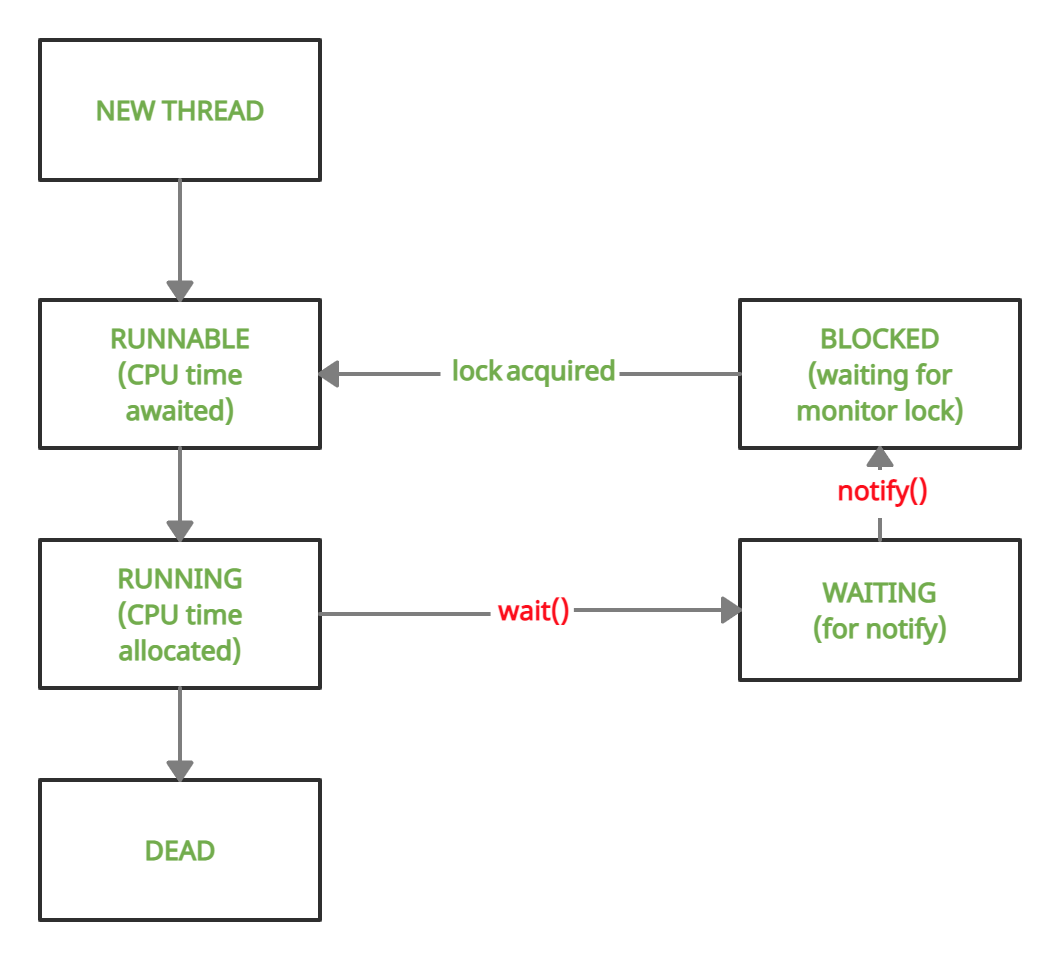Open in App
Not now

# Difference Between wait() and notify() in Java

• Difficulty Level : Medium
• Last Updated : 04 Apr, 2022

The wait() and notify() are methods of the Object class. They were introduced to part ways with polling, which is the process of repeatedly checking for a condition to be fulfilled. Polling wastes CPU resources considerably, hence it is not preferred.

### wait() Method

wait() method is a part of java.lang.Object class. When wait() method is called, the calling thread stops its execution until notify() or notifyAll() method is invoked by some other Thread.

The wait() method has 3 variations:

1. wait(): This is a basic version of the wait() method which does not take any argument. It will cause the thread to wait till notify is called.

`public final void wait()`

2. wait(long timeout): This version of the wait() method takes a single timeout argument. It will cause the thread to wait either till notify is called or till timeout (One which occurs earlier).

`public final void wait(long timeout)`

3. wait(long timeout, int nanoseconds): This version of the wait() method takes a timeout argument as well as a nanosecond argument for extra precision.

`public final void wait(long timeout, int nanoseconds)`

### notify() Method

The notify() method is defined in the Object class, which is Java’s top-level class. It’s used to wake up only one thread that’s waiting for an object, and that thread then begins execution. The thread class notify() method is used to wake up a single thread.

`public final void notify()`### Differences between wait() and notify()

Below is demonstration of wait() and notify() method:

## Java

 `// Java Program to demonstrate usage of wait() and notify()` `class` `demo {``    ``// variable to check if part1 has returned``    ``// volatile used to prevent threads from``    ``// storing local copies of variable``    ``volatile` `boolean` `part1done = ``false``;` `    ``// method synchronized on this``    ``// i.e. current object of demo``    ``synchronized` `void` `part1()``    ``{``        ``System.out.println(``"Welcome to India"``);``        ``part1done = ``true``;``        ``System.out.println(``            ``"Thread t1 about to surrender lock"``);``        ``// notify the waiting thread, if any``        ``notify();``    ``}` `    ``// method synchronized on this``    ``// i.e. current object of demo``    ``synchronized` `void` `part2()``    ``{``        ``// loop to prevent spurious wake-up``        ``while` `(!part1done) {``            ``try` `{``                ``System.out.println(``"Thread t2 waiting"``);``                ``// wait till notify is called``                ``wait();``                ``System.out.println(``                    ``"Thread t2 running again"``);``            ``}``            ``catch` `(Exception e) {``                ``System.out.println(e.getClass());``            ``}``        ``}``        ``System.out.println(``"Do visit Taj Mahal"``);``    ``}``}` `public` `class` `Main {` `    ``public` `static` `void` `main(String[] args)``    ``{` `        ``// Make an instance of demo class``        ``demo obj = ``new` `demo();` `        ``// Thread t1 will call part1()``        ``Thread t1 = ``new` `Thread(``new` `Runnable() {``            ``public` `void` `run() { obj.part1(); }``        ``});` `        ``// Thread t2 will call part2()``        ``Thread t2 = ``new` `Thread(``new` `Runnable() {``            ``public` `void` `run() { obj.part2(); }``        ``});` `        ``// Start t2 and then t1``        ``t2.start();``        ``t1.start();``    ``}``}`

Output

```Thread t2 waiting
Welcome to India
Do visit Taj Mahal```

### Various Exceptions:

1. wait()

• It is mandatory to enclose wait() in a try-catch block because if a thread present in the waiting state gets interrupted, then it will throw InterruptedException.
• The other two variations of wait housing parameters will throw IllegalArgumentException if the value of timeout is negative or the value of nanoseconds is not in the range 0 to 9,99,999.

Below is the implementation for the exception handling.

## Java

 `// Program demonstrating occurrence of InterruptedException` `class` `demo {``    ``volatile` `boolean` `part1done = ``false``;` `    ``synchronized` `void` `part1()``    ``{``        ``System.out.println(``"Welcome to India"``);``        ``part1done = ``true``;``        ``// notify() has been commented, waiting``        ``// thread remains waiting forever notify();``    ``}` `    ``synchronized` `void` `part2()``    ``{``        ``while` `(!part1done) {``            ``try` `{``                ``wait();``            ``}``            ``catch` `(Exception e) {``                ``System.out.println(``"Exception : "``                                   ``+ e.getClass());``                ``// quit program after exception is thrown``                ``System.exit(-``1``);``            ``}``        ``}``        ``System.out.println(``"Do visit Taj Mahal"``);``    ``}``}` `public` `class` `Main {` `    ``public` `static` `void` `main(String[] args)``    ``{``        ``// Make an instance of demo class``        ``demo obj = ``new` `demo();` `        ``// Thread t1 will call part1()``        ``Thread t1 = ``new` `Thread(``new` `Runnable() {``            ``public` `void` `run() { obj.part1(); }``        ``});` `        ``// Thread t2 will call part2()``        ``Thread t2 = ``new` `Thread(``new` `Runnable() {``            ``public` `void` `run() { obj.part2(); }``        ``});` `        ``// Start t2 and then t1``        ``t2.start();``        ``t1.start();` `        ``// This is a counter which will``        ``// interrupt Thread t2 after 3 seconds``        ``long` `startTime = System.currentTimeMillis();``        ``while` `(``true``) {``            ``if` `(System.currentTimeMillis() - startTime``                ``> ``3000``)``                ``t2.interrupt();``        ``}``    ``}``}`

Output

```Welcome to India
Exception : class java.lang.InterruptedException```

2. notify()

Unlike wait(), the notify method does not throw an InterruptedException hence it is not mandatory to house it inside a try-catch block

Note:

• wait() and notify() both have a tendency to throw IllegalMonitorStateException
• This occurs when a thread is holding the monitor lock of object A and tries to call wait or notify on object B.
• In all the preceding examples, the methods were synchronized on “this” i.e. the object used to call those methods (obj). Also, the wait() & notify() were being called as this.wait() and this.notify() (usage of this was redundant). Hence, there was no issue.
• In the below example, the methods part1 and part2 are now synchronized on an Integer object, but wait() & notify() are still being called on the object which called these methods (obj).
• This causes an IllegalMonitorStateException

Below is the implementation of the exception handling.

## Java

 `// Program to demonstrate IllegalMonitorStateException` `class` `demo {``    ``volatile` `boolean` `part1done = ``false``;``    ``// Made an Integer object a``    ``// and set it randomly to 5``    ``Integer a = ``5``;` `    ``void` `part1()``    ``{``        ``// Synchronized code on a``        ``synchronized` `(a)``        ``{``            ``System.out.println(``"Welcome to India"``);``            ``part1done = ``true``;``            ``// calling this.notify()``            ``notify();``        ``}``    ``}` `    ``void` `part2()``    ``{``        ``// Synchronized code on a``        ``synchronized` `(a)``        ``{``            ``while` `(!part1done) {``                ``try` `{``                    ``// calling this.wait()``                    ``wait();``                ``}``                ``catch` `(Exception e) {``                    ``System.out.println(``"Exception: "``+e.getClass());``                    ``System.exit(-``1``);``                ``}``            ``}``            ``System.out.println(``"Do visit Taj Mahal"``);``        ``}``    ``}``}` `public` `class` `Main {` `    ``public` `static` `void` `main(String[] args)``    ``{` `        ``// Make an instance of demo class``        ``demo obj = ``new` `demo();` `        ``// Thread t1 will call part1()``        ``Thread t1 = ``new` `Thread(``new` `Runnable() {``            ``public` `void` `run() { obj.part1(); }``        ``});` `        ``// Thread t2 will call part2()``        ``Thread t2 = ``new` `Thread(``new` `Runnable() {``            ``public` `void` `run() { obj.part2(); }``        ``});` `        ``// Start t2 and then t1``        ``t2.start();``        ``t1.start();``    ``}``}`

Output

`Exception: class java.lang.IllegalMonitorStateException`

Note: To fix the above code, just replace notify() with a.notify() on line 17 and wait() with a.wait() on line 29.

My Personal Notes arrow_drop_up